##### Page tree

Should you require any more information or have encountered a problem, please call the support helpdesk on (07) 5456 6000.

Go to start of banner

# Using a library and functions

## Overview

GELLO allows the creation of Libraries and functions. A library is a collection of non volatile resources which in this case is for the consistent implementation and reuse of code. It is useful to tuck away snippets of code for commonly used methods or to hide the complexity of large and occasionally used code. This tutorial will demonstrate two examples of the former, one of the latter and then bring them together by way of worked examples.

## Calculate patient age

In an earlier tutorial we wrote GELLO to calculate the age of a patient in years from the VMR instance's date of birth. Now we will convert that code into a function in a Library called TestLib. Open the GELLO Editor and in a fresh workspace write:

`  Package TestLib  imports HL7_v2_VMR_V1`

`  EndPackage`

Compile and save as TestLib.gello_model

(Make sure you haven't saved it as TestLib.model. I suggest you write or cut and paste the whole file name including the extension in the File name box after choosing Save As from the menu.)

Now add at about line 4 ( making sure we are adding to the GELLO above the EndPackage line):

`  --functions`
`  GetAgeInYears(vmr:SinglePatient): PQ =                               Let dob: TS = vmr.patient.dob                               Let ageInSeconds: PQ = factory.TS('today') - dob                               Let ageInYrs: PQ = ageInSeconds.convert('yr')                               Let ageInYrsRoundedDown: PQ = factory.PQ(ageInYrs.value.floor(),'yr')                                   in                               ageInYrsRoundedDown`

Compile and save.

So here is our first function in TestLib. The function name is capitalised. We have added a floor() method on the value of the age, made the patient class explicitly derived from the vmr,  and used a line containing the word 'in'. The reason for the 'in' is that a GELLO expression is intrinsically made up of an inner expression and an outer expression with the 'in' linking them.

In previous code in earlier tutes the editor has said we need a final declarative line. When that is added, that was in fact the outer expression. The code above it was the inner expression and the 'in' was not mandatory- but it could have been added.

Ok now lets write some GELLO that makes use of this library:

In a new workspace (hit the New button on top left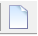), put:

`     imports HL7_v2_VMR_V1, TestLib     Context SinglePatient`
`     Let age: PQ = GetAgeInYears(Self)`
`     age`

and run with fourthTest.xml as the data: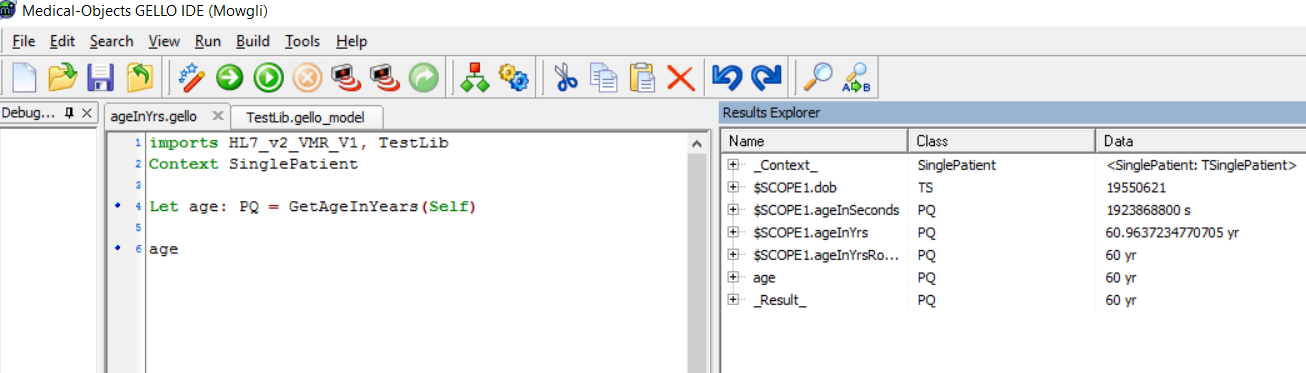The TestLib library is imported in the first line. The function needs a parameter called Self.

Note: the gello file and the gello_model Library file need to be in the same folder when saved on your machine.

## Calculate BMI

Now for a similarly sized function to add to the Library. This one calculates Body Mass index (BMI) for a patient, making use of the patient's most recent height and weight measurements. We will need to add the following data to our test file and then save it as fifthTest.xml .

<vitals>
<!-- weight is an Observation-->
<weight>
<observationCode code="27113001"
codeSystem="2.16.840.1.113883.6.96"
codeSystemName="SNOMED-CT">
<displayName value = "body weight" />
<translation code="3141-9"
codeSystem="2.16.840.1.113883.6.1"
codeSystemName="LN">
<displayName value = "Body weight Measured" />
</translation>
</observationCode>
<dateTime value = "20160423" />
<value xsi:type = "PQ" value = "68" unit = "kg" />
</weight>
<weight>
<observationCode code="27113001"
codeSystem="2.16.840.1.113883.6.96"
codeSystemName="SNOMED-CT">
<displayName value = "body weight" />
<translation code="3141-9"
codeSystem="2.16.840.1.113883.6.1"
codeSystemName="LN">
<displayName value = "Body weight Measured" />
</translation>
</observationCode>
<dateTime value = "20101102" />
<value xsi:type = "PQ" value = "66" unit = "kg" />
</weight>
<height>
<observationCode code="50373000"
codeSystem="2.16.840.1.113883.6.96"
codeSystemName="SNOMED-CT">
<displayName value = "body height" />
<translation code=" 8308-9"
codeSystem="2.16.840.1.113883.6.1"
codeSystemName="LN">
<displayName value = "Body height Measured" />
</translation>
</observationCode>
<dateTime value = "20101102" />
<value xsi:type = "PQ" value = "164" unit = "cm" />
</height>
</vitals>

We have two weights from different dates and a height measurement.

The formula for BMI is Bodyweight in kilograms divided by height in meters squared.

Add this to the functions section of the Library file and save.

`    GetBMI(vmr:SinglePatient): Real =      Let latestHtObservation: Observation = vmr.vitals.height->sortedBy(dateTime)->last()      Let latestHt_PQ: PQ = latestHtObservation.value.oclAsType(PQ).convert('m')      Let latestWt: PQ = vmr.vitals.weight->sortedBy(dateTime)->last().value.oclAsType(PQ)      Let bmi: Real = latestWt.value/latestHt_PQ.value.power(2)       in      bmi`

We are getting the latest height and weight observations and then getting the value out as PQs.

Here's how it should look: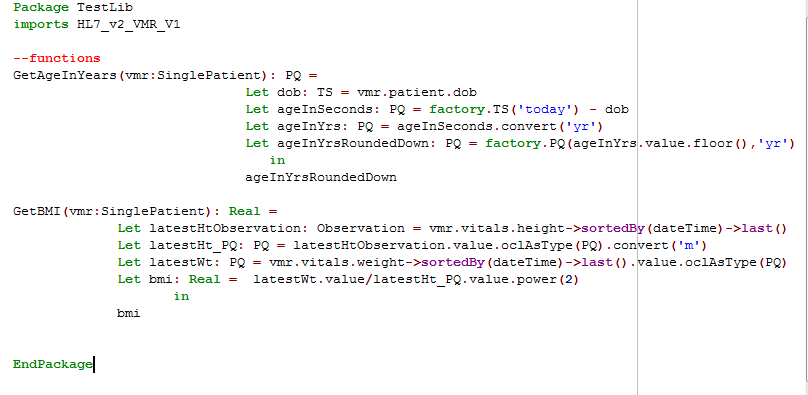and here is some GELLO code to call it:

`    imports HL7_v2_VMR_V1, TestLib    Context SinglePatient`
`    Let bmi: Real = GetBMI(Self)    bmi`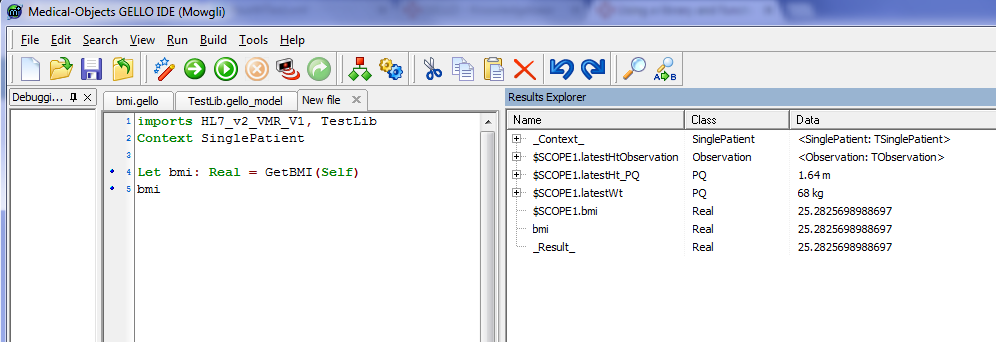## First degree relatives example

Ok so now we will demonstrate a complex multi-lined function extending the work we did earlier on family history. It turns out there are lots of SNOMED CT codes for first, second and third degree relatives; so it makes sense to not have to write all these out every time we want to find say all first degree relatives and their clinical genomic choices for a given patient.

Our test data says the patient has seven relatives - how many are first degree?

Open the file TestLib.gello_model again.

Make some room above the '-- functions' line.

Insert the following:

`    --Let statements for SCT family history concepts    Let naturalFather_SCT_Concept : CD = CD{code='9947008',codeSystem = '2.16.840.1.113883.6.96',codeSystemName ='SNOMED-CT'}    Let father_SCT_Concept : CD = CD{code='66839005',codeSystem = '2.16.840.1.113883.6.96',codeSystemName ='SNOMED-CT'}    Let fatherOfSubject_SCT_Concept : CD = CD{code='444295003',codeSystem = '2.16.840.1.113883.6.96',codeSystemName ='SNOMED-CT'}    Let naturalSon_SCT_Concept : CD = CD{code='113160008',codeSystem = '2.16.840.1.113883.6.96',codeSystemName ='SNOMED-CT'}    Let son_SCT_Concept : CD = CD{code='65616008',codeSystem = '2.16.840.1.113883.6.96',codeSystemName ='SNOMED-CT'}    Let sonOfSubject_SCT_Concept : CD = CD{code='444241008',codeSystem = '2.16.840.1.113883.6.96',codeSystemName ='SNOMED-CT'}    Let naturalBrother_SCT_Concept : CD = CD{code='60614009',codeSystem = '2.16.840.1.113883.6.96',codeSystemName ='SNOMED-CT'}    Let brother_SCT_Concept : CD = CD{code='70924004',codeSystem = '2.16.840.1.113883.6.96',codeSystemName ='SNOMED-CT'}    Let brotherOfSubject_SCT_Concept : CD = CD{code='444303004',codeSystem = '2.16.840.1.113883.6.96',codeSystemName ='SNOMED-CT'}    Let twinBrother_SCT_Concept : CD = CD{code='81276006',codeSystem = '2.16.840.1.113883.6.96',codeSystemName ='SNOMED-CT'}    Let fraternalTwinBrother_SCT_Concept : CD = CD{code='81467001',codeSystem = '2.16.840.1.113883.6.96',codeSystemName ='SNOMED-CT'}    Let identicalTwinBrother_SCT_Concept : CD = CD{code='78194006',codeSystem = '2.16.840.1.113883.6.96',codeSystemName ='SNOMED-CT'}`

These are all the first degree male relatives in the Person hierarchy of SNOMED CT. Add the following to bring them together in a set:

`    Let FDR_male_relatives:Set(CD) = Set{ naturalFather_SCT_Concept, father_SCT_Concept, fatherOfSubject_SCT_Concept,     naturalSon_SCT_Concept, son_SCT_Concept, sonOfSubject_SCT_Concept, naturalBrother_SCT_Concept, brother_SCT_Concept, brotherOfSubject_SCT_Concept, twinBrother_SCT_Concept,  fraternalTwinBrother_SCT_Concept,     identicalTwinBrother_SCT_Concept}`

Compile to check there are no cut and paste errors and save. Now add the same structure for female first degree relative concepts:

`    Let naturalMother_SCT_Concept : CD = CD{code='65656005',codeSystem = '2.16.840.1.113883.6.96',codeSystemName ='SNOMED-CT'}    Let mother_SCT_Concept : CD = CD{code='72705000',codeSystem = '2.16.840.1.113883.6.96',codeSystemName ='SNOMED-CT'}    Let motherOfSubject_SCT_Concept : CD = CD{code='444301002',codeSystem = '2.16.840.1.113883.6.96',codeSystemName ='SNOMED-CT'}    Let naturalDaughter_SCT_Concept : CD = CD{code='83420006',codeSystem = '2.16.840.1.113883.6.96',codeSystemName ='SNOMED-CT'}    Let daughter_SCT_Concept : CD = CD{code='66089001',codeSystem = '2.16.840.1.113883.6.96',codeSystemName ='SNOMED-CT'}    Let daughterOfSubject_SCT_Concept : CD = CD{code='444194006',codeSystem = '2.16.840.1.113883.6.96',codeSystemName ='SNOMED-CT'}    Let naturalSister_SCT_Concept : CD = CD{code='73678001',codeSystem = '2.16.840.1.113883.6.96',codeSystemName ='SNOMED-CT'}    Let sister_SCT_Concept : CD = CD{code='27733009',codeSystem = '2.16.840.1.113883.6.96',codeSystemName ='SNOMED-CT'}    Let sisterOfSubject_SCT_Concept : CD = CD{code='444304005',codeSystem = '2.16.840.1.113883.6.96',codeSystemName ='SNOMED-CT'}    Let twinSister_SCT_Concept : CD = CD{code='19343003',codeSystem = '2.16.840.1.113883.6.96',codeSystemName ='SNOMED-CT'}    Let fraternalTwinSister_SCT_Concept : CD = CD{code='29644004',codeSystem = '2.16.840.1.113883.6.96',codeSystemName ='SNOMED-CT'}    Let identicalTwinSister_SCT_Concept : CD = CD{code='50058005',codeSystem = '2.16.840.1.113883.6.96',codeSystemName ='SNOMED-CT'}`
`    Let FDR_female_relatives:Set(CD) = Set{ naturalMother_SCT_Concept, mother_SCT_Concept, motherOfSubject_SCT_Concept,     naturalDaughter_SCT_Concept, daughter_SCT_Concept, daughterOfSubject_SCT_Concept,     naturalSister_SCT_Concept, sister_SCT_Concept, sisterOfSubject_SCT_Concept,     twinSister_SCT_Concept, fraternalTwinSister_SCT_Concept, identicalTwinSister_SCT_Concept}`

In the functions section, above 'EndPackage', add the following:

`    SelectedRelatives(relatives: Sequence(Relative), matches: Set(CD)): Sequence(Relative) =     relatives->select(x | matches->includes(x.relationship))`
`    FDR_male(familyHistory: FamilyHistory): Sequence(Relative) =     SelectedRelatives(familyHistory.relatives, FDR_male_relatives)`
`    FDR_female(familyHistory: FamilyHistory): Sequence(Relative) =     SelectedRelatives(familyHistory.relatives, FDR_female_relatives)`

These functions take the relationship CDs we have made, looks for them in the family history for the patient and then returns either the male first degree relatives or the female first degree relatives as we might specify. (Looking for male first degree relatives  can matter if we are interested in a gender specific illness such as prostate cancer.)

Ok lets try it out. Save our newly expanded TestLib.gello_model and in a new window write:

`     imports HL7_v2_VMR_V1, TestLib     Context SinglePatient       Let fdr_female:Sequence(Relative) = FDR_female(familyHistory)     Let fdr_male: Sequence(Relative) = FDR_male(familyHistory)       Let firstDegreeRelatives: Sequence(Relative) = fdr_female->union(fdr_male)     firstDegreeRelatives`

Running this against fifthTest.xml should give us three first degree relatives (you may need to save the gello_model file and the new gello files in the same folder and close and then reopen the editor; if you get nulls):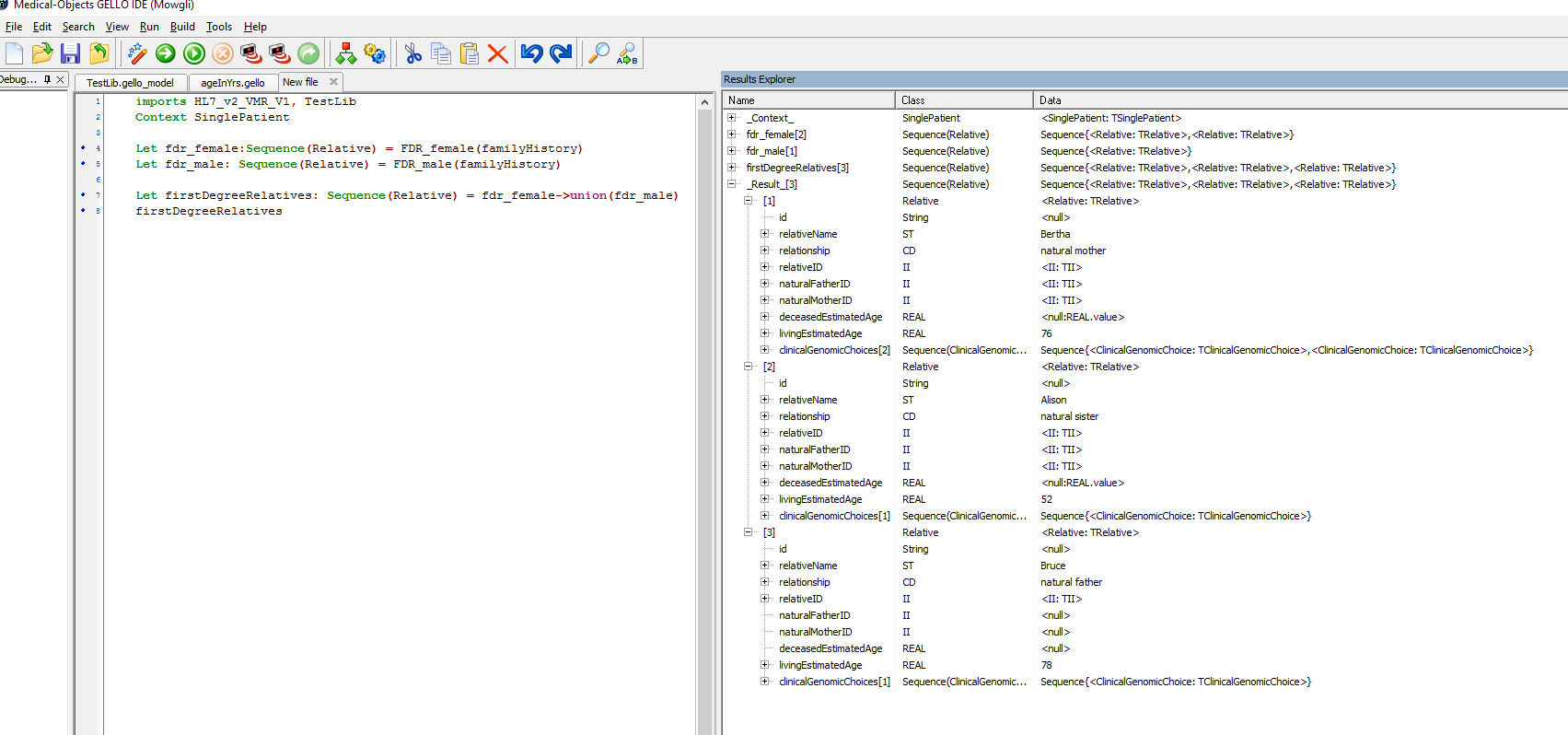## Some code to use all three examples in one

Ok to finish off here is some code using all of our Functions in the Test Library:

Lets say we wanted to know if the patient, was underweight, under fifty years of age and has a first degree relative with colon cancer. (This is an example and we wouldn't rely on these criteria in the real world - but sadly rates of colon cancer in young people are on the rise)

`    imports HL7_v2_VMR_V1, TestLib`
`    Context SinglePatient      --underweight?     Let patient_bmi : Real = GetBMI(Self)    Let isUnderweight: Boolean = patient_bmi <= 18.5`
`    -- under fifty?    Let isUnderFifty: Boolean = GetAgeInYears(Self).value < 50`
`    --FDR with colon cancer?    Let fdr_female:Sequence(Relative) = FDR_female(familyHistory)    Let fdr_male: Sequence(Relative) = FDR_male(familyHistory)     Let firstDegreeRelatives: Sequence(Relative) = fdr_female->union(fdr_male)    Let colonCancer: CD = factory.CD_SNOMED('363406005','malignant tumor of colon')    Let fdrWithColonCa: Boolean = firstDegreeRelatives ->     select(clinicalGenomicChoices -> select(clinicalObservation.implies(colonCancer).value            and (not negationIndicator.value))->notEmpty())->notEmpty()`
`    --final result     isUnderweight and     isUnderFifty and      fdrWithColonCa`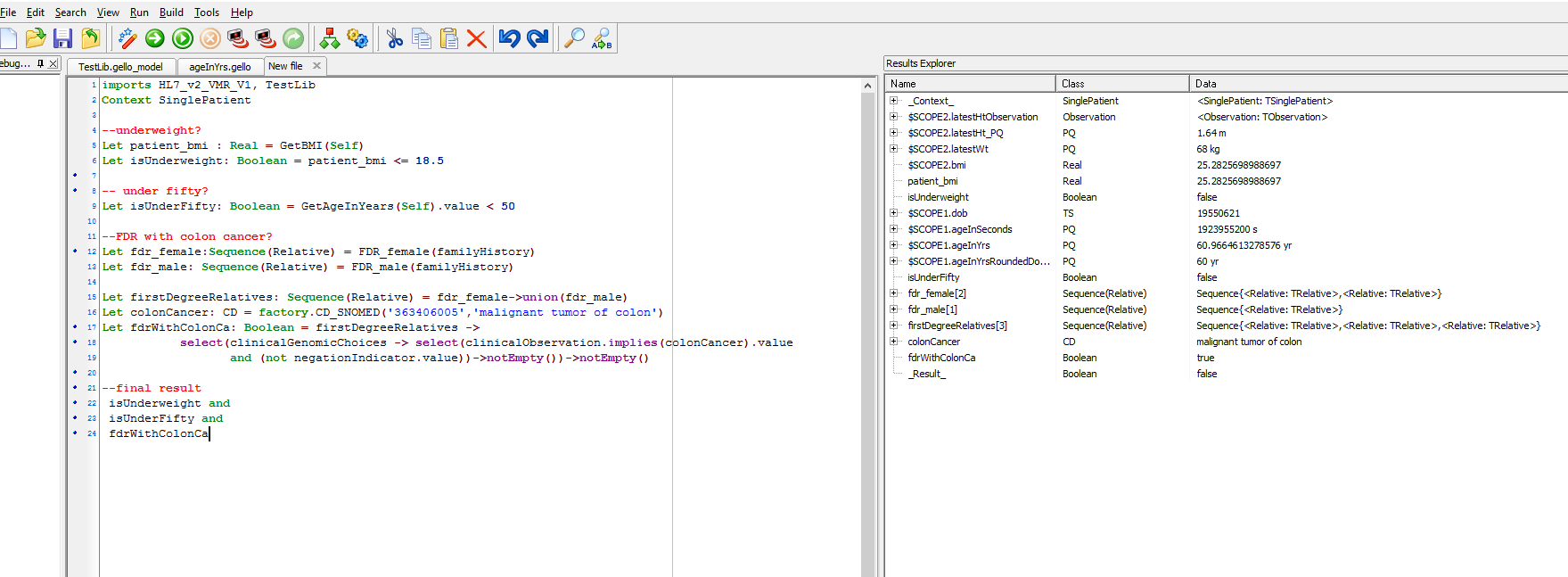Remember the functions in this example are those starting with a capital letter. So in the result we see this patient has one of the three criteria and so overall we return a False value.

That's the end of this tutorial.

• No labels